• Call Now

1800-102-2727•

Assume in a class of 20 students, all the students are bright, intelligent and well-mannered. Another 10 students are admitted to this class from the outside. They are rather distracting, arrogant, and uninterested in their studies. The introduction of 10 students from the outside has now degraded the overall performance of the class.Those 20 students are analogues to an ideal solution with no deviations, but the 10 students admitted from outside are non-ideal solutions with more deviations.

An ideal solution is a mixture in which distinct species of molecules can be differentiated; however, unlike the ideal gas, the molecules in the ideal solution exert forces on one another. When such forces come out to be the same for all molecules independent of species, then a solution is considered to be ideal.

• Definition of a Solution
• Types of Solutions
• What is an Ideal solution?
• Characteristics of Ideal Solutions
• Graphical Representation of an Ideal Solution
• Examples of Ideal Solutions
• What is a Non-Ideal Solution?
• Characteristics of Non-Ideal Solutions
• Graphical Representation of a Non-Ideal Solution
• Examples of Non-Ideal Solutions
• Practice Problems
• Frequently Asked Questions - FAQs

Definition of Solution

A solution is a homogenous mixture of two or more components. It is a mixture which has a consistent composition throughout. A homogeneous solution is also known as a true solution.

Types of Solutions

There are various types of solutions that can be classified based on how the solute and solvent are used.

Based on the use of water as a solvent:

• Aqueous solution: An aqueous solution is created by the dissolution of a solute in water. Examples of aqueous solutions include water-based solutions of glucose, sodium chloride, and soap.
• Non-Aqueous solution: When a solute is dissolved in a liquid that is not water, such as benzene,acetone, ethanol, carbon disulfide, and so on, the result is a non-aqueous solution.

Based on the quantity of solvent added:

• Concentrated solution: In a concentrated solution, there is a significant amount of the solute in the provided solvent. Examples include black tea, apple juice, and brine solution.
• Dilute solution: A diluted solution contains a greater proportion of solvent.

Based on the quantity of solute added:

• Saturated solution: In a saturated solution, the solute is entirely dissolved in the solvent at the specified temperature.
• Unsaturated solution: A solution that allows for more solute dissolution at the same temperature is said to be unsaturated.
• Supersaturated solution: When a solute starts to precipitate out after being dissolved at a specific concentration and temperature, the solution is said to be supersaturated.

Based on the amount of concentration of solute in two solutions:

• Isotonic solution: The term "isotonic solution" refers to two solutions with the same osmotic pressure.
• Hypertonic solution: The term "hypertonic solution" refers to a solution that has a higher osmotic pressure than another solution.
• Hypotonic solution: The term "hypotonic solution" refers to a solution that has a lower osmotic pressure than the another solution.

What is an Ideal Solution?

Ideal solutions are those solutions that obey Raoult's law  over the entire concentration range.

Characteristics of Ideal Solutions

Suppose there are two different pure liquids,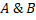, which then mixed together. Now, if these two together form an ideal solution, following are the characteristics of this solution:

•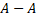interactions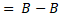interactions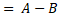interactions
•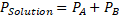•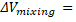0
•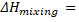0
•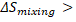0
• Δ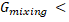0

Graphical Representation of an Ideal Solution

A plot of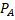and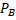versus the mole fractions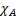and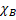for a solution gives a linear plot as shown in the figure below. These lines pass through the points for which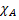andare equal to unity. Similarly, the plot of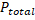versusis also linear as shown in the figure below. The maximum value ofis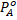and the minimum value is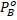assuming that the component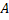is more volatile than the component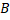,i.e.,>.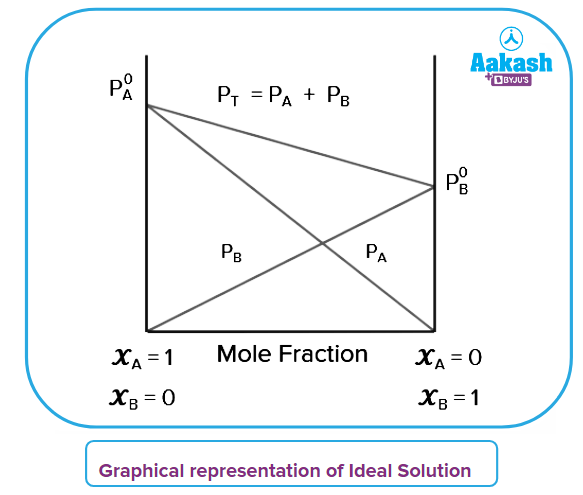Examples of Ideal Solutions

Following are solutions which behave like ideal solutions:

•  Ethyl bromide + Ethyl iodide
•  Ethanol + Methanol
•  Chlorobenzene + Bromobenzene
•  n-Butyl chloride + n-Butyl bromide
•  n-Hexane + n-Heptane

What is a Non-Ideal Solution?

A non-ideal solution is one that does not obey Raoult's law throughout the whole concentration range.

There are two types of non-ideal solutions:

• Non-ideal solutions shows positive deviation
• Non-ideal solution shows negative deviation

Characteristics of Non-Ideal Solutions

Suppose there are two different pure liquids,, which then mixed together. Now, if these two together form a non-ideal solution, following are the characteristics of this solution:

•interactions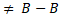interactions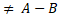interactions
•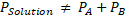•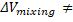0
•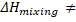0
•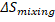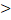•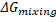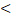0

Characteristics of non-ideal solution showing positive deviation:

If the total vapour pressure is higher than that calculated from Raoult’s law then it is called a non-ideal solution showing positive deviation.

•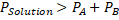• The interactions between solute-solvent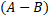is weaker than those of pure components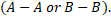•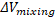•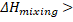•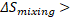•0

Characteristics of non-ideal solution showing negative deviation:

If the total vapour pressure of the solution is lower than that of which calculated from Raoult’s law then it is called a non-ideal solution which is showing negative deviation.

•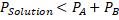• The interactions between solute-solventis stronger than those of pure components••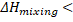••0

Graphical Representation of a Non-Ideal Solution

Non-Ideal solution showing positive deviation:

The total vapour pressure of the solution exceeds that predicted by Raoult's equation as shown below by plotting a graph between vapour pressure and mole fraction.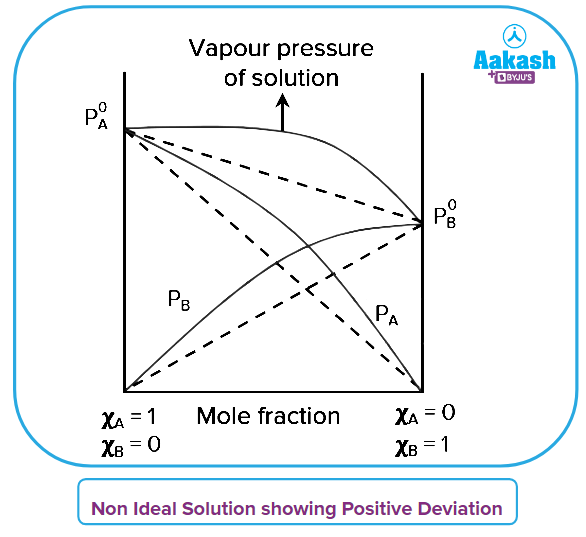Non-Ideal solution showing negative deviation:

The total vapour pressure of the solution is less than that predicted by Raoult's equation as shown below by plotting a graph between vapour pressure and mole fraction.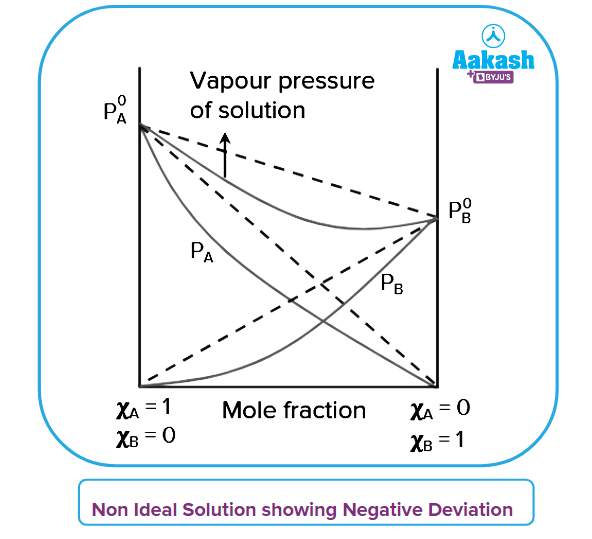Examples of Non-ideal Solutions

Examples of solutions showing positive deviation:

• Acetone + Ethanol
• Acetone + Carbon disulphide
• Water + Ethanol
• Carbon Tetrachloride + Toluene
• Acetone + Benzene
• Carbon tetrachloride + Methanol
• Water + Methanol
• Cyclohexane + Ethanol

Examples of solutions showing negative deviation:

• Acetone + Aniline
• Methanol + Acetic acid
• Chloroform + diethyl ether
• Acetic acid + Pyridine
• Chloroform + Benzene
• Water + Nitric acid

Practice Problems

Q.1. When a solution of two liquids is boiled at a temperature more than the boiling point of either of them, the binary solution will show:

(A) no deviation from Raoult’s law
(B) negative deviation from Raoult’s law
(C) positive deviation from Raoult’s law
(D) None of the above

Solution: Solutions boil at comparatively greater temperatures because the vapour pressure of the solution will be less than that of pure components. It demonstrates a negative deviation from Raoult's law and strong interaction between the solute and the solvent (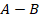) than that of pure components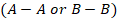.

Q.2. Which of the following forms an ideal solution?

(A) n-butyl bromide and n-butyl iodide
(B) Acetone and Ethanol
(C) Water and Ethanol
(D) Chloroform and Benzene

Solution: For a solution to be ideal in nature, the solute-solute interaction and solvent-solvent interaction should be almost similar to the solute-solvent interaction. Among the given options, only n-butyl bromide and n-butyl iodide show such interactions. Hence, they form an ideal solution.

Q.3. The change in gibbs free energy for an ideal solution is:

(A)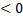(B)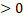(C)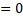(D) Can not defined

Solution: We can calculate the change in gibbs free energy of a system which is defined as the enthalpy of the system minus the product of the temperature times the entropy change of the system.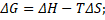For ideal solutions, the value of the gibbs free energy change comes out to be negative as mixing of ideal solutions is a spontaneous process.

Q.4. Which of the following observations about the interaction between acetone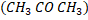and chloroform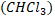is true?

(A) Compared to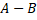interactions,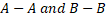interactions are stronger.
(B) Compared tointeractions,interactions are weaker.
(C)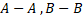and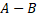interactions are all equivalent.
(D) The liquids separate into distinct layers and are immiscible.

Solution: A mixture of acetone () and chloroform (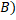results in a solution () that deviates negatively from Raoult's law. This is due to the ability of the chloroform molecule to form a hydrogen bond with the acetone molecule. It will display a negative deviation becauseand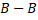interactions are weaker than theinteractions.

Q.1. What are miscible and immiscible liquids?

Answer: Two liquids that are completely dissolved in each other are called miscible liquids. For example, water and ethanol are the best pair of miscible liquids.

Two liquids that are not completely dissolved with each other are called immiscible liquids. For example, water and petrol are the best pair of immiscible liquids.

Q.2. Explain, in terms of intermolecular forces, why water and octane are not miscible with each other?

Answer: While octane molecules interact through London interactions, water molecules interact through hydrogen bonds i.e., dipole-dipole interactions. So, when we try to mix octane with water, there are no interaction between the water and octane molecules.

Q.3. What type of intermolecular attractions are present between the pair n-hexane and n-octane?

Answer: Since both the compounds are alkanes, their mixture has van der Waals forces of attraction between them and the mixture of these two will form an ideal solution.

Q.4. What are the examples of a solvent and a solute?

Answer: The substance that typically determines the physical state of the solution is the solvent (solid, liquid or gas). The product that the solvent dissolves is known as the solute. For example, in a solution of salt and water, the solute is salt and the solvent is water.

Related Topics:Talk to our expert
Resend OTP Timer =
By submitting up, I agree to receive all the Whatsapp communication on my registered number and Aakash terms and conditions and privacy policy